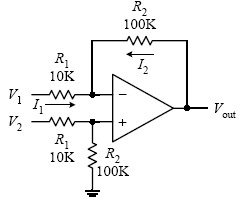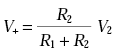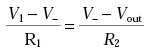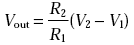Home | Gadget | Fuzzy | Lecturer  | Power  | Transceiver Find more type here... Difference Amplifier byDetermine Vout. Need to process two signal using math function as difference use this circuit. Difference signal almost as first method in sensor and instrument amplifier.First, you determine the voltage at the noninverting input by using the voltage divider relation (again, assume that no current enters the inputs):Next, apply Kirchhoff’s current junction law to the inverting input (I1 = I2):Using rule 4 (V+ = V−), substitute the V+ term in for V− in the last equation to getIf you set R1 = R2, then Vout = V2 − V1. This material comes from any source reference and the copyright is own the author him self not this sites Copyright 2009 (c) technosains.com  All Rights Reserved